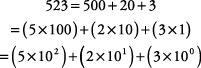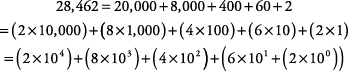## Place Value

Our number system is a place value system; that is, each place is assigned a different value. For instance, in the number 675, the 6 is in the hundreds place, the 7 is in the tens place, and the 5 is in the ones place. Because the number system is based on powers of 10 (10 0 = 1, 10 1 = 10, 10 2 = 10 × 10 = 100, 10 3 = 10 × 10 × 10 = 1,000, and so on), each place is a progressive power of ten, as shown in Table 1.

Table 1. Place value systemNotice how the number 675 fits into the place value grid.

Sometimes, numbers are written in expanded notation to point out the place value of each digit.

##### Example 1

Write 523 in expanded notation.These last two are the more common forms of expanded notation: one without exponents, one with exponents. Notice that in these the digit is multiplied times its place value: 1's, 10's, 100's, and so on.

##### Example 2

Write 28,462 in expanded notation.#### Rounding off

To round off any number,

Underline the place value to which you're rounding off.

Look to the immediate right (one place) of your underlined place value.

Identify the number (the one to the right). If it is 5 or higher, round your underlined place value up 1 and change all the other numbers to its right to zeros. If the number (the one to the right) is 4 or less, leave your underlined place value as it is and change all the other numbers to its right to zeros.

##### Example 3

Round off 345,678 to the nearest thousand.

The number 34 5,678 is rounded up to 346,000.

##### Example 4

Round off 724,591 to the nearest ten thousand.

The number 7 24,591 is rounded down to 720,000.

Numbers that have been rounded off are called rounded numbers.